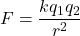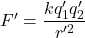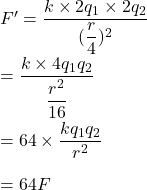## What happens to the force between two charges when each charge is doubled and the distance between them is 1/4 its original magnitude?

Question

What happens to the force between two charges when each charge is doubled and the distance between them is 1/4 its original
magnitude?

in progress 0
2 weeks 2021-09-05T14:18:53+00:00 1 Answers 0 views 0

F’ = 64 F

Explanation:

The electric force between charges is given by :Where

q₁ and q₂ are charges

r is the distance between charges

When  each charge is doubled and the distance between them is 1/4 its original magnitude such that,

q₁’ = 2q₁, q₂’ = 2q₂ and r’ = (r/4)

New force,Apply new values,So, the new force becomes 64 times the initial force.